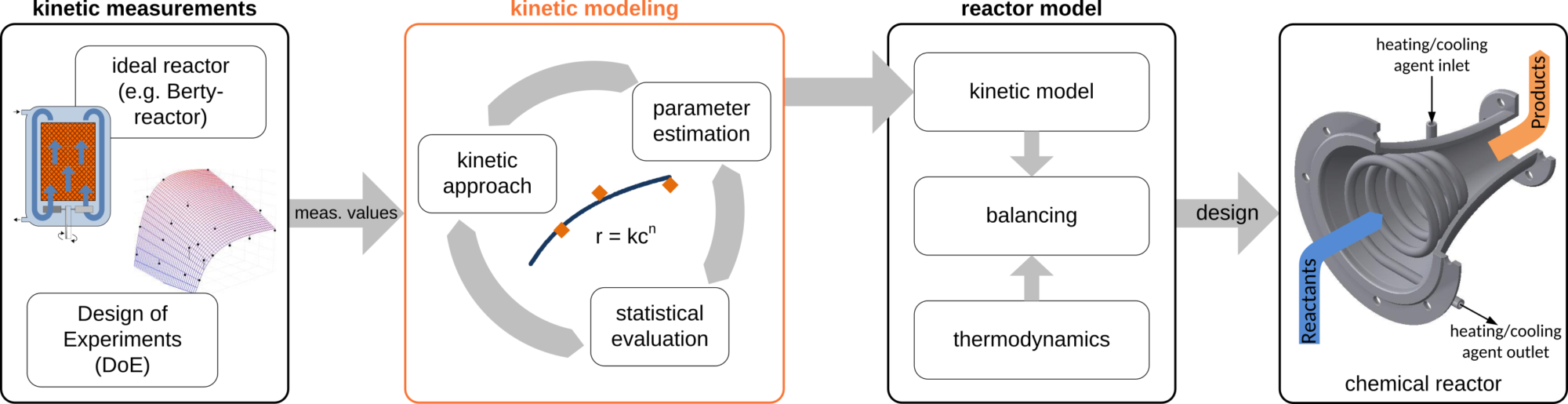# Kinetic Modelling

## Modeling of reaction kinetics

Process optimization in chemical industries requires precise knowledge of the considered reaction system, which may consist of one or more reaction steps. Such knowledge allows for the development of new or enhancement of existing processes regarding economics, safety, efficiency etc. Therefore, the design and optimization of the chemical reactor as core element of the process is of particular interest. Generally reactor design is based on balance equations, which contain thermodynamic and kinetic models. A kinetic model constitutes the mathematical description of the course of the reaction for each reaction step as a function of components in the system.

First of all, the development of a kinetic model requires measurements in an ideal laboratory scale reactor. To keep the total number of experiments reasonable and to perform the experiments well adapted to the sensitivity of the regarded system, measurements are organized via statistical methods in a “Design of Experiments” (DoE). Taking the gathered data as a basis, suitable kinetic approaches that rely on mechanistic assumptions (of different level of detail) with a qualitatively similar course of data points are then selected for kinetic modeling. Besides state variables (e.g., concentration or temperature) kinetic approaches contain fitting parameters, which may be varied to adapt the result of the mathematical approach to the measured values (e.g., activation energy, pre-exponential factor). In order to identify the most suitable and reliable kinetic approach for each reaction step, the fitting results are evaluated by means of statistical analysis (confidence intervals, correlation coefficients, residuals, etc.). Accordingly, the final result of the kinetic modeling is a fully parametrized model to describe each reaction step of the reaction network. The Institute of Chemical Reaction Engineering provides the possibility to perform kinetic measurements and kinetic modeling. For this, several continuous and batch laboratory scale reactors are available, which allow different parameters to be varied and whose setup is customizable to various requirements. For kinetic modeling diverse state-of-the-art software tools (e.g. Presto Kinetics, Aspen Custom Modeler, Comsol Multiphyics) are in use.Fig. 1: Kinetic modeling as part of chemical reactor design.

### Example:

Reactant A reacts via two intermediate products B and C to the final product D. Possible reaction networks might look like as follows:Fig. 2: Linear reaction network (left), branched reaction network (right)

For the branched example from Figure 2, the set of differential equations, which comprises the kinetic model, can be written in the following way:Fig. 3: Kinetic model

As described earlier, a suitable kinetic approach has to be identified for each reaction step. Power law approaches are well known and simple. They characterize the course of reaction by a temperature dependent rate constant k(T) and the components concentration or partial pressure, respectively. There are numerous kinetic approaches available, of which the most common are depicted in Figure 4.Fig. 4: Examples for kinetic expression

Typically, the rate constant k(T) is calculated using the Arrhenius expression:Here, EA and k0 represent the activation energy and the frequency factor of the reaction.

### Fundings and Cooperations

Industry partners